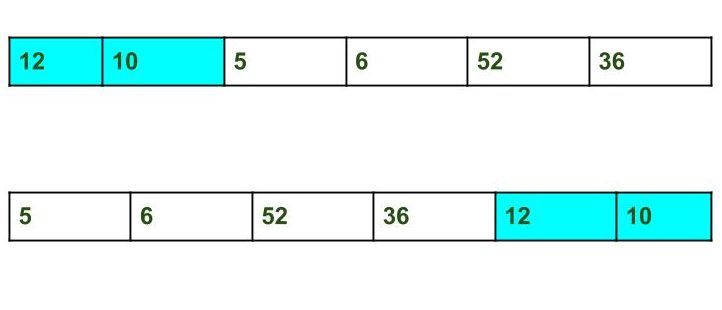Skip to content
Related Articles
Split the array and add the first part to the end | Set 2
• Difficulty Level : Easy
• Last Updated : 16 Jun, 2021

Given an array and split it from a specified position, and move the first part of array add to the end.Examples:

```Input : arr[] = {12, 10, 5, 6, 52, 36}
k = 2
Output : arr[] = {5, 6, 52, 36, 12, 10}
Explanation : Split from index 2 and first
part {12, 10} add to the end .

Input : arr[] = {3, 1, 2}
k = 1
Output : arr[] = {1, 2, 3}
Explanation : Split from index 1 and first
part add to the end.```

A O(n*k) solution is discussed here
This problem can be solved in O(n) time using the reversal algorithm discussed below,
1. Reverse array from 0 to n – 1 (where n is size of the array).
2. Reverse array from 0 to n – k – 1.
3. Reverse array from n – k to n – 1.

## C++

 `// C++ program to Split the array and``// add the first part to the end``#include ` `using` `namespace` `std;` `/* Function to reverse arr[] from index start to end*/``void` `rvereseArray(``int` `arr[], ``int` `start, ``int` `end)``{``    ``while` `(start < end) {``        ``int` `temp = arr[start];``        ``arr[start] = arr[end];``        ``arr[end] = temp;``        ``start++;``        ``end--;``    ``}``}` `// Function to print an array``void` `printArray(``int` `arr[], ``int` `size)``{``    ``for` `(``int` `i = 0; i < size; i++)``        ``cout << arr[i] << ``" "``;``}` `/* Function to left rotate arr[] of size n by k */``void` `splitArr(``int` `arr[], ``int` `k, ``int` `n)``{``    ``rvereseArray(arr, 0, n - 1);``    ``rvereseArray(arr, 0, n - k - 1);``    ``rvereseArray(arr, n - k, n - 1);``}` `/* Driver program to test above functions */``int` `main()``{``    ``int` `arr[] = { 12, 10, 5, 6, 52, 36 };``    ``int` `n = ``sizeof``(arr) / ``sizeof``(arr);``    ``int` `k = 2;` `    ``// Function calling``    ``splitArr(arr, k, n);``    ``printArray(arr, n);` `    ``return` `0;``}`

## Java

 `// Java program to Split the array and``// add the first part to the end``class` `Geeks``{``    ` `/* Function to reverse arr[] from index start to end*/``static` `void` `rvereseArray(``int` `arr[], ``int` `start, ``int` `end)``{``    ``while` `(start < end) {``        ``int` `temp = arr[start];``        ``arr[start] = arr[end];``        ``arr[end] = temp;``        ``start++;``        ``end--;``    ``}``}` `// Function to print an array``static` `void` `printArray(``int` `arr[], ``int` `size)``{``    ``for` `(``int` `i = ``0``; i < size; i++)``        ``System.out.print(arr[i] +``" "``);``}` `/* Function to left rotate arr[] of size n by k */``static` `void` `splitArr(``int` `arr[], ``int` `k, ``int` `n)``{``    ``rvereseArray(arr, ``0``, n - ``1``);``    ``rvereseArray(arr, ``0``, n - k - ``1``);``    ``rvereseArray(arr, n - k, n - ``1``);``}` `/* Driver program to test above functions */``public` `static` `void` `main(String args[])``{``    ``int` `arr[] = { ``12``, ``10``, ``5``, ``6``, ``52``, ``36` `};``    ``int` `n = arr.length;``    ``int` `k = ``2``;` `    ``// Function calling``    ``splitArr(arr, k, n);``    ``printArray(arr, n);` `}` `}` `// This code is contributed by ankita_saini.`

## Python3

 `# Python3 program to Split the array``# and add the first part to the end` `# Function to reverse arr[]``# from index start to end*/``def` `rvereseArray(arr, start, end):``    ``while` `start < end :``        ``temp ``=` `arr[start]``        ``arr[start] ``=` `arr[end]``        ``arr[end] ``=``temp``        ``start ``+``=` `1``        ``end ``-``=` `1` `# Function to print an array``def` `printArray(arr, n) :``    ``for` `i ``in` `range``(n):``        ``print``(arr[i], end ``=` `" "``)`  `# Function to left rotate``# arr[] of size n by k */``def` `splitArr(arr, k, n):``    ``rvereseArray(arr, ``0``, n ``-` `1``)``    ``rvereseArray(arr, ``0``, n ``-` `k ``-` `1``)``    ``rvereseArray(arr, n ``-` `k, n ``-` `1``)` `# Driver Code``arr ``=` `[``12``, ``10``, ``5``, ``6``, ``52``, ``36``]``n ``=` `len``(arr)``k ``=` `2``splitArr(arr, k, n)``printArray(arr, n)` `# This code is contributed``# by Shrikant13`

## C#

 `// C# program to Split the array and``// add the first part to the end``using` `System;``class` `GFG``{``    ` `/* Function to reverse arr[] from index start to end*/``static` `void` `rvereseArray(``int``[] arr, ``int` `start, ``int` `end)``{``    ``while` `(start < end)``    ``{``        ``int` `temp = arr[start];``        ``arr[start] = arr[end];``        ``arr[end] = temp;``        ``start++;``        ``end--;``    ``}``}` `// Function to print an array``static` `void` `printArray(``int``[] arr, ``int` `size)``{``    ``for` `(``int` `i = 0; i < size; i++)``        ``Console.Write(arr[i] +``" "``);``}` `/* Function to left rotate arr[] of size n by k */``static` `void` `splitArr(``int``[] arr, ``int` `k, ``int` `n)``{``    ``rvereseArray(arr, 0, n - 1);``    ``rvereseArray(arr, 0, n - k - 1);``    ``rvereseArray(arr, n - k, n - 1);``}` `// Driver code``public` `static` `void` `Main()``{``    ``int``[] arr = { 12, 10, 5, 6, 52, 36 };``    ``int` `n = arr.Length;``    ``int` `k = 2;` `    ``// Function calling``    ``splitArr(arr, k, n);``    ``printArray(arr, n);``}``}` `// This code is contributed``// by Akanksha Rai(Abby_akku)`

## PHP

 ``

## Javascript

 ``

Output:

`5 6 52 36 12 10 `

Attention reader! Don’t stop learning now. Get hold of all the important DSA concepts with the DSA Self Paced Course at a student-friendly price and become industry ready.  To complete your preparation from learning a language to DS Algo and many more,  please refer Complete Interview Preparation Course.

In case you wish to attend live classes with industry experts, please refer DSA Live Classes

My Personal Notes arrow_drop_up